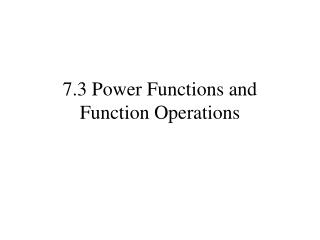DownloadDownload Presentation7.3 Power Functions and Function Operations

# 7.3 Power Functions and Function Operations

Télécharger la présentation## 7.3 Power Functions and Function Operations

- - - - - - - - - - - - - - - - - - - - - - - - - - - E N D - - - - - - - - - - - - - - - - - - - - - - - - - - -
##### Presentation Transcript

1. 7.3 Power Functions and Function Operations

2. Objectives/Assignment • Perform operations with functions including power functions • Assignment: 13-51 odd

3. In chapter 6 you learned how to add, subtract, multiply, and divide polynomial functions. These operations can be defined for any function. The domain of h consists of the x-values that are in the domains of both f and g. Additionally, the domain of a quotient does not include x-values for which g(x) = 0.

4. So far you have studied various types of functions, including linear functions, quadratic functions, and polynomial functions of higher degree. Another common type of function is a power function: Where a is a real number and b is a rational number. Notice when b =1, f(x) is a linear function. when b=2, f(x) is a quadratic function. when b=3, f(x) is a cubic function.

5. Here is another way to write the composition of f and g: